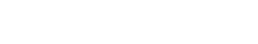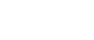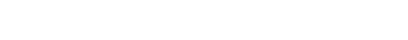# Calculate Quantities of Materials for Concrete -Cement, Sand, AggregatesReading time: 1 minute

Quantities of materials for concrete such as cement, sand and aggregates for production of required quantity of concrete of given mix proportions such as 1:2:4 (M15), 1:1.5: 3 (M20), 1:1:2 (M25) can be calculated by absolute volume method. This method is based on the principle that the volume of fully compacted concrete is equal to the absolute volume of all the materials of concrete, i.e. cement, sand, coarse aggregates and water.A Concrete structure may consists of beams, slabs, columns and foundations etc. based on type of structure. The volume of concrete required for concrete structure can be calculated by summing up the volumes of each structural member or each parts of members.

The volume of a rectangular cross sectional member can be calculated as length x width x height (or depth or thickness). Suitable formula shall be used for different cross-sectional shapes of members.

The formula for calculation of materials for required volume of concrete is given by:Where, Vc = Absolute volume of fully compacted fresh concrete W =Mass of water C = Mass of cement Fa = Mass of fine aggregates Ca = Mass of coarse aggregates Sc, Sfa and Sca are the specific gravities of cement, fine aggregates and coarse aggregates respectively. The air content has been ignored in this calculation. This method of calculation for quantities of materials for concrete takes into account the mix proportions from design mix or nominal mixes for structural strength and durability requirement. Now we will learn the material calculation by an example.

## Calculating Quantities of Materials for per cubic meter or cubic feet or cubic yards concrete

Consider concrete with mix proportion of 1:1.5:3 where, 1 is part of cement, 1.5 is part of fine aggregates and 3 is part of coarse aggregates of maximum size of 20mm. The water cement ratio required for mixing of concrete is taken as 0.45.

Assuming bulk densities of materials per cubic meter, cubic feet and cubic yards as follows: Cement = 1500 kg/m3 = 93.642 lb/ft3 = 2528.332 lb/cubic yards Sand = 1700 kg/m3 = 105 lb/ft3 = 2865.443 lb/cubic yards Coarse aggregates = 1650 kg/m3 = 103 lb/ft3 = 2781.166 lb/cubic yards Specific gravities of concrete materials are as follows: Cement = 3.15 Sand = 2.6 Coarse aggregates = 2.6. The percentage of entrained air assumed is 2%. The mix proportion of 1:1.5:3 by dry volume of materials can be expressed in terms of masses as: Cement = 1 x 1500 = 1500 Sand = 1.5 x 1700 = 2550 Coarse aggregate = 3 x 1650 = 4950. Therefore, the ratio of masses of these materials w.r.t. cement will as follows == 1 : 1.7 : 3.3

The water cement ratio = 0.45 Now we will calculate the volume of concrete that can be produced with one bag of cement (i.e. 50 kg cement) for the mass proportions of concrete materials. Thus, the absolute volume of concrete for 50 kg of cement =Thus, for the proportion of mix considered, with one bag of cement of 50 kg, 0.1345 m3 of concrete can be produced. We have considered an entrained air of 2%. Thus the actual volume of concrete for 1 cubic meter of compacted concrete construction will be = 1 -0.02 = 0.98 m3. Thus, the quantity of cement required for 1 cubic meter of concrete = 0.98/0.1345 = 7.29 bags of cement. The quantities of materials for 1 m3 of concrete production can be calculated as follows: The weight of cement required = 7.29 x 50 = 364.5 kg. Weight of fine aggregate (sand) = 1.5 x 364.5 = 546.75 kg. Weight of coarse aggregate = 3 x 364.5 = 1093.5 kg.

## Concrete Calculator: Calculate Quantities of Materials for Concrete

 Select Concrete Mix Proportion Cement : Sand : Aggregates : W/C Ratio :
 Select Concrete Member*: Select Options Slab Footing Rectangular Column Circular Column Beam
 Dimension of Concrete Member Length /Height: mm Width : mm Thickness / Depth : mm Diameter : mm

## Calculation Results: Quantities of Materials

Volume of Concrete = m3 = Yards = CFT = Pounds

Quantity of Cement = kg = Bags of 50 kg = Pounds

Quantity of Sand = kg = m3 = Yards = CFT = Pounds

Quantity of Coarse Aggregates = kg = m3 = Yards = CFT = Pounds

Quantity of Water = Liter = Gallons